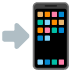# Easiest way to map 0 to 360 degrees with 0 at 12 o’clock

I want to make an interface for rotation when mouseX, mouseY are rotated around a circle it will output 0 - 360 degrees with 0 being at 12 o’clock. I have tried a few ways to do this but it’s not working.

This is the closest I have gotten but it puts 0 degrees at 9 o’clock.

Can someone please help me? At the map function I’d like angleDeg to be assigned 0-360 degrees, with 0 becoming the value when the line is at 12 o’clock.

I’ve been searching the forums and the internet for a few days and can not find an explanation of how to do this.

``````void draw() {

PVector mouse = new PVector(mouseX, mouseY);
PVector center = new PVector(width/2, height/2);
mouse.sub(center);

angleDeg = map(degrees(angleRad), -180, 180, 0, 360);
println(angleDeg);

mouse.normalize();
mouse.mult(100);

pushMatrix();
translate(width/2, height/2);
line(0, 0, mouse.x, mouse.y);
popMatrix();
}
``````

Hi ddownn,

You want the values to increase clockwise, so 9 o’clock is 270 degrees, right? Floor modulo (as opposed to truncation modulo, the `%`) may help you simplify the angle values you receive from `heading` or `atan2`, since it takes the negative values out of the calculation. Since 0 degrees rotation is 3 o’clock, you have to take an extra `HALF_PI` (90 degrees) into account.``````void draw() {
background(255);
PVector mouse = new PVector(mouseX, mouseY);
PVector center = new PVector(width * 0.5, height * 0.5);
PVector diff = PVector.sub(mouse, center);

float angleDeg = degrees(floorMod(angleRad + HALF_PI, TWO_PI));

pushMatrix();
translate(center.x, center.y);
stroke(127);
line(0, 0, 100, 0);
fill(0);
text(angleDeg, 15, 0);
popMatrix();
}

static float floorMod(float a, float b) {
return a - b * floor(a / b);
}
``````

Hope that helps!

1 Like

Yes, it really does help, thank you. And thanks for the link. It’s going to take me a while to wrap my mind around why it works, but at least I know where to start. I knew I had to do something with HALF_PI.

Couldn’t you also add 90 degrees to the angle to “convert” it to “0 degrees is 12:00” space?

That’s the first thing I tried, adding 90 degrees counts from 0 at 12:00 to 270 at 9:00, but leaves 9:00 to 12:00 counting down from -90 to 0.
Then I tried the map function to get a continuous 0-360 count, but couldn’t find a way to change the 0 position.

``````void setup() {
size(500, 500);
}

void draw() {
background(255);
PVector mouse = new PVector(mouseX, mouseY);
PVector center = new PVector(width * 0.5, height * 0.5);
PVector diff = PVector.sub(mouse, center);

//angleDeg = degrees(floorMod(angleRad + HALF_PI, TWO_PI));

pushMatrix();
translate(center.x, center.y);
stroke(127);
line(0, 0, 100, 0);
fill(0);
textSize(24);
text(angleDeg, 25, 0);
popMatrix();
}
a = degrees(a) + 90;
return a;
}
float mapFirst(float a) {
a = map(degrees(a), -180, 180, 0, 360);
return a;
}

static float floorMod(float a, float b) {
return a - b * floor(a / b);
}
``````

It means floorMod() can be directly called by other classes w/o needing to instantiate the `class` which floorMod() method belongs to.It also means method floorMod() can’t directly access any non-`static` members of its own `class`.